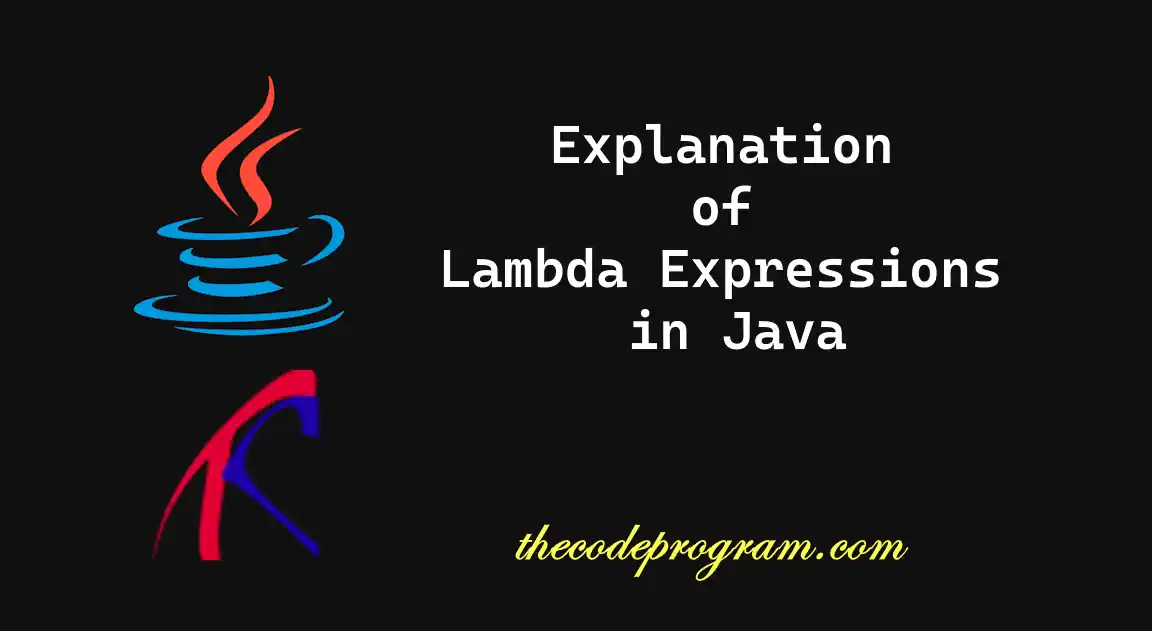## Explanation of Lambda Expressions in Java

Hello everyone, in this article we are going to talk about Lambda Expressions in Java and how to use it to help the improving the code readability and maintainability.

Let's get started.

Lambda expressions are introduced with Java 8 and lead to a new way to write simpler code in Java. With Lambda expressions, we can create more functionality with and expressive.

#### What Are Lambda Expressions?

Lambda expressions allow us to write methods as functional-style in Java. We can use these functions to express entire result of another function or the function itself. Stream methods in Java is one of the best example of lambda expressions in Java.

Below you can see the example syntax of Lambda expressions in Java.
``````
(parameters) -> expression
``````
In above definition
• parameters: Are parameters for the expression. If there is no parameter just use empty parenthesis '()'.
• ->: The arrow token is separating the parameters and expression.
• expression: Specifies. the expression body. If it is single line you can directly use, if it is a function we enclose the code block with braces '{}'.

#### Now let's make some examples.

We can see Lambda expressions with stream functions of the lists in Java.
``````
import java.util.*;

class LambdaInStream {
public static void main(String[] args) {
List<Integer> numbers = Arrays.asList(1, 2, 3, 4, 5, 6, 7, 8, 9, 10);

numbers.stream()
.filter(n -> n % 2 == 0)
.map(n -> Integer.toString(n))
.forEach(n -> {
System.out.println(n);
});
}
}
``````
Program output will be like below
``````
2
4
6
8
10
``````

As you can see above we have used the lambda expressions in filter, map and forEach functions. In filter and map we used a single expression and in forEach we used a normal method body.

We can define functional interfaces. We can define an interface with one abstract method as you can see below. Then we will asign the inline code(lambda expression) to this interface. In in future when we call this abstract method, our lambda expression will be invoked.

``````
interface LambdaInterface
{
//definition of abstract method
void proceed(int x);
}

class HelloWorld {
public static void main(String[] args) {
// Definition of our lambda function
LambdaInterface fobj = (int x) -> System.out.println(x*x);

// This calls above lambda expression and prints 10.
fobj.proceed(3);
}
}
``````
Program output will be
``````
9
``````

As you can see above we have initialised the Functional interface with an inline method and when we invoked the abstract method, our custom method is invoked.

We can also use the functional interface under the java.util.function package. We can define our lambda expressions and calling these functions directly.

``````
import java.util.function.*;
import java.util.*;

class HelloWorld {
public static void main(String[] args) {

//Example of Consumer
List<String> words = Arrays.asList("the", "code", "program");
Consumer<String> print = (word) -> System.out.println(word);
words.forEach(print);

//Example of Function
Function<String, String> toUpperCase = (word) -> word.toUpperCase();
words
.stream()
.map(toUpperCase)
.forEach(System.out::println);

//Example of Predicate
Predicate<String> isLongWord = (word) -> word.length() > 5;
words
.stream()
.filter(isLongWord)
.forEach(System.out::println);

//Example of Supplier
Supplier<String> currentDate = () -> new Date().toString();
System.out.println(currentDate.get());
}
}
``````
Program output will be like below
``````
the
code
program
THE
CODE
PROGRAM
program
Thu Oct 05 19:32:55 GMT 2023
``````

As you can see above we have tested some of the examples of functional interfaces with lambda expressions. Below you can see the definitions of them. For more functional interfaces please check the official Java Documentation

There are more functional interface under the package. Here is the basic definitions of our examples.
• Consumer takes an argument and performs an action but doesn't return a value
• Function takes an argument and returns a result.
• Supplier takes no arguments and returns a value.
• Predicate takes an argument and returns a boolean value.

That is all.

Burak Hamdi TUFAN

23/12/2019

09/07/2021